RRB NTPC Mock Test - 15 (English)

# RRB NTPC Mock Test - 15 (English)

Test Description

## 100 Questions MCQ Test RRB NTPC - Mock Tests Papers 2020 | RRB NTPC Mock Test - 15 (English)

RRB NTPC Mock Test - 15 (English) for Railways 2022 is part of RRB NTPC - Mock Tests Papers 2020 preparation. The RRB NTPC Mock Test - 15 (English) questions and answers have been prepared according to the Railways exam syllabus.The RRB NTPC Mock Test - 15 (English) MCQs are made for Railways 2022 Exam. Find important definitions, questions, notes, meanings, examples, exercises, MCQs and online tests for RRB NTPC Mock Test - 15 (English) below.
Solutions of RRB NTPC Mock Test - 15 (English) questions in English are available as part of our RRB NTPC - Mock Tests Papers 2020 for Railways & RRB NTPC Mock Test - 15 (English) solutions in Hindi for RRB NTPC - Mock Tests Papers 2020 course. Download more important topics, notes, lectures and mock test series for Railways Exam by signing up for free. Attempt RRB NTPC Mock Test - 15 (English) | 100 questions in 90 minutes | Mock test for Railways preparation | Free important questions MCQ to study RRB NTPC - Mock Tests Papers 2020 for Railways Exam | Download free PDF with solutions
 1 Crore+ students have signed up on EduRev. Have you?
RRB NTPC Mock Test - 15 (English) - Question 1

### A cylinder of height 15 cm and base diameter 14 cm is cut from a paper sheet which is in parallelogram shape with base 50 cm and height 18 cm. Find the area of the remaining sheet. Use π = 22/7.

Detailed Solution for RRB NTPC Mock Test - 15 (English) - Question 1

⇒ Area of parallelogram paper sheet = base × height = 50 × 18 = 900 cm2

Now,

⇒ Area of sheet cut for cylinder = Curved surface area of cylinder = 2π × base radius × height = 2 × (22/7) × (14/2) × 15 = 660 cm2

⇒ Area of remaining sheet = 900 – 660 = 240 cm2

RRB NTPC Mock Test - 15 (English) - Question 2

### The mean of 15 numbers is 40. If two numbers 45 and 35 are removed, then what will be the mean of remaining numbers?

Detailed Solution for RRB NTPC Mock Test - 15 (English) - Question 2

Average of 15 numbers is 40

∴ Sum of these 15 numbers = 15 × 40 = 600

If two numbers 45 and 35 are removed

Sum of remaining 13 numbers = 600 - 45 - 35 = 520

∴ Average of these 13 numbers = 520/13 = 40

RRB NTPC Mock Test - 15 (English) - Question 3

### In a certain code, RANDOM is written as XFRGQN, then can be DANCER written as

Detailed Solution for RRB NTPC Mock Test - 15 (English) - Question 3

We observe the following pattern: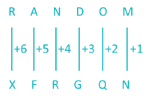Hence using the same pattern for DANCER: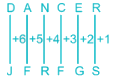RRB NTPC Mock Test - 15 (English) - Question 4

Select a suitable figure from the answer figures that would replace the question mark (?)Detailed Solution for RRB NTPC Mock Test - 15 (English) - Question 4

As ​the mouse is a device controlled using hands, the headset is a device for the ears.

Hence, option 2 is the correct answer.

RRB NTPC Mock Test - 15 (English) - Question 5

Which of the given fractions is NOT equal to 9/17?

Detailed Solution for RRB NTPC Mock Test - 15 (English) - Question 5

Option 1: 27/51 = 9/17

Option 2: 63/119 = 9/17

Option 3: 153/289 = 9/17

Option 4: 108/221 ≠ 9/17

∴ 108/221 is NOT equal to 9/17.

RRB NTPC Mock Test - 15 (English) - Question 6

Where is the headquarters of South Eastern Central Railway zone located?

Detailed Solution for RRB NTPC Mock Test - 15 (English) - Question 6
• The South East Central Railway's (SECR) headquarters is located in Bilaspur.
• It is among the 17 Indian Rail zones.
• It was previously under the control of the South Eastern Railway.
RRB NTPC Mock Test - 15 (English) - Question 7

________ are used for communication in artificial satellites.

Detailed Solution for RRB NTPC Mock Test - 15 (English) - Question 7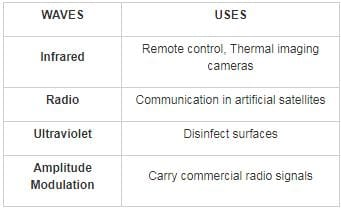RRB NTPC Mock Test - 15 (English) - Question 8

Arrange the following options in logical order.

1. Click on the compose button to create new message.
2. Press the maximize button on New message to expand the window.
3. Fill the From and To field.
5. Fill the Subject field.
6. Attach any files if needed and Click on Send.

Detailed Solution for RRB NTPC Mock Test - 15 (English) - Question 8

The given options show how to compose and send a new message in Gmail.

1. Click on the compose button to create new message.

2. Press the maximize button on New message to expand the window.

3. Fill the From and To field.

5. Fill the Subject field.

6. Attach any files if needed and Click on Send.

Thus, 412356 is the correct order.

RRB NTPC Mock Test - 15 (English) - Question 9

Choose the pair which is related in the same way as the words in the first pair from the given choices

Praise : Admonish : ---------- : -----------

Detailed Solution for RRB NTPC Mock Test - 15 (English) - Question 9

Admonish is the opposite of Praise. Therefore, we need to choose antonyms.

Tough : Crunchy

Clearly not antonyms

Attractive : Tempting

They are synonyms, not antonyms

Dull : Bright

Antonyms of each other.

Tame : Trained

They are synonyms, not antonyms

Hence, Dull : Bright is the correct answer.

RRB NTPC Mock Test - 15 (English) - Question 10

If A : B = 3 : 4 and B : C = 4 : 5, then what is A : B : C?

Detailed Solution for RRB NTPC Mock Test - 15 (English) - Question 10

A : B = 3 : 4

Let A = 3x and B = 4x

B : C = 4 : 5

Let B = 4x and C = 5x

A : B : C = 3x : 4x : 5x = 3 : 4 : 5

RRB NTPC Mock Test - 15 (English) - Question 11

Two bodies are in thermal equilibrium, if they have the same ________.

Detailed Solution for RRB NTPC Mock Test - 15 (English) - Question 11

Two physical systems are in thermal equilibrium if there is no net flow of thermal energy between them when they are connected by a path permeable to heat. Thermal equilibrium obeys the zeroth law of thermodynamics.

RRB NTPC Mock Test - 15 (English) - Question 12

Which among the following is known as the richest mineral belt in India?

Detailed Solution for RRB NTPC Mock Test - 15 (English) - Question 12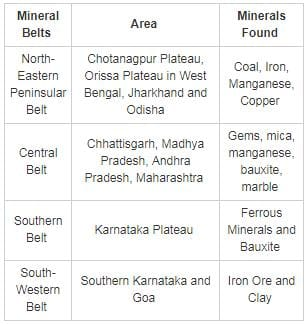RRB NTPC Mock Test - 15 (English) - Question 13

The average age of a family of 4 members in the year 2005 was 20. A new member was born in the same family in 2005. Find the average age of the family on the 6th birthday of the youngest member.

Detailed Solution for RRB NTPC Mock Test - 15 (English) - Question 13

The average age of a family of 4 members in the year 2005 was 20

Since the age of the youngest member in 2005 is 0, the total age of all 5 members of the family in the year 2005 = 4 x 20 + 0 = 80

Total age of the family after 6 years will be = total age now + 5 x 6 = 80 + 30 = 110

Average age of the family then = 110/ 5= 22

RRB NTPC Mock Test - 15 (English) - Question 14

Background image or text in a document is known as ________.

Detailed Solution for RRB NTPC Mock Test - 15 (English) - Question 14

A watermark is a logo or text superimposed on an image to help prevent an image from being copied or allow others to know where it was copied from and who owns the rights. Watermarks are often used on web pages so that the site's logo or banner is always visible in the background. A digital watermark is a kind of marker covertly embedded in a noise-tolerant signal such as an audio, video or image data. It is typically used to identify ownership of the copyright of such signal.

RRB NTPC Mock Test - 15 (English) - Question 15

Which of the following phenomena makes endoscopy possible?

Detailed Solution for RRB NTPC Mock Test - 15 (English) - Question 15

Total internal reflection is a phenomenon in optics in which a light ray reaching a medium boundary at greater than the critical angle is completely reflected in the same medium. An endoscope is an instrument used by doctors to examine the inside of the body. It consists of a bundle of optical fibres so as to transmit the image of organs around the corners.

RRB NTPC Mock Test - 15 (English) - Question 16

Chamera Dam is situated in which among the following states in India?

Detailed Solution for RRB NTPC Mock Test - 15 (English) - Question 16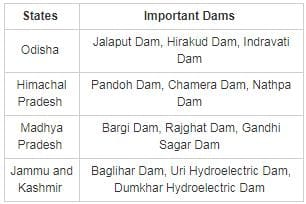RRB NTPC Mock Test - 15 (English) - Question 17

Study the following information carefully and answer the given questions.

In a certain code language ‘mat is wet’ is written as ‘fuss do rah’, ‘car is red’ is written as ‘el ne do’, ‘yellow mat’ is written as ‘fuss no’ and ‘violet car’ is written as ‘ne phi’. How is ‘wet yellow car’ written in that code language?

Detailed Solution for RRB NTPC Mock Test - 15 (English) - Question 17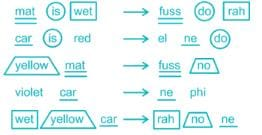‘wet’ is coded as ‘rah’ and ‘yellow’ is coded as ‘no’ and ‘car’ is coded as ‘ne’.

Hence, “wet yellow car” is coded as “rah no ne”.

RRB NTPC Mock Test - 15 (English) - Question 18

Ability to distinguish two closely placed objects is ______.

Detailed Solution for RRB NTPC Mock Test - 15 (English) - Question 18

Resolving Power:

• The ability of an optical instrument or type of film to separate or distinguish small or closely adjacent images
• Of the human eye with 20/20 vision is typically considered to be about “one arc minute” or “60 arc seconds”
RRB NTPC Mock Test - 15 (English) - Question 19

In the following question, select the related letters / numbers from the given alternatives.

ALPHA : 0116 : : BETA : ?

Detailed Solution for RRB NTPC Mock Test - 15 (English) - Question 19

Logic:

The first two digits of the number is the minimum number value among all the alphabetical values of the given alphabets.

The last two digits of the number is the maximum number value among all the alphabetical values of the given alphabets.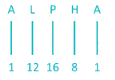Minimum among 1, 12, 16, 8 and 1 is 1. Maximum among 1, 12, 16, 8 and 1 is 16.

So, ALPHA is written as 0116.

Similarly,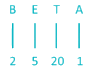Minimum among 2, 5, 20 and 1 is 1. Maximum among 2, 5, 20 and 1 is 20.

So, BETA is written as 0120.

Hence, “0120” is the correct answer from the given alternatives.

RRB NTPC Mock Test - 15 (English) - Question 20

A can build a hall measuring 12 ft × 5 ft × 15 ft in 3 days and B can build a hall in 25 ft × 16 ft × 10 ft in 8 days. What is the ratio of the work done by A and B in one day?

Detailed Solution for RRB NTPC Mock Test - 15 (English) - Question 20

⇒ One day work of A = (12 ft × 5 ft × 15 ft)/3 = 300 ft

⇒ One day work of B = (25 ft × 16 ft × 10 ft)/8 = 500 ft

∴ Ratio of efficiency = 300 ∶ 500 = 3 ∶ 5

RRB NTPC Mock Test - 15 (English) - Question 21

Which game has 7 players in each side?

Detailed Solution for RRB NTPC Mock Test - 15 (English) - Question 21
• Water polo, consisting of 6 field players and 1 goal keeper and Kabaddi are the sports which have 7 players each in a team.
• On the other hand, basketball has 5 players in each side and Baseball has 9 players.
RRB NTPC Mock Test - 15 (English) - Question 22

In the following question, select the related word from the given alternatives.
India : Men In Blue : : New Zealand : ?

Detailed Solution for RRB NTPC Mock Test - 15 (English) - Question 22

As the Indian National men’s cricket team is also known as “Men in Blue”, similarly the New Zealand National men’s cricket team is also known as “Black Caps”

Hence, “Black Caps” is the correct answer from the given alternatives.

RRB NTPC Mock Test - 15 (English) - Question 23

Directions: In each question below is given a statement followed by two assumptions numbered I and II. You have to consider the statements and the following assumptions and decide which of the assumptions is implicit in the statement.

Statement: “Computer education should start in primary schools itself.”

Assumptions:
I. Learning computers is easy.
II. Computer education can increase the chances of getting a job.

Detailed Solution for RRB NTPC Mock Test - 15 (English) - Question 23

Clearly, computer education can be started at the school level only if it is easy. So, I is implicit. In the statement, nothing is mentioned about the link between jobs and computer education. So, II is not implicit.

RRB NTPC Mock Test - 15 (English) - Question 24

The rubbing surface of a matchbox has powdered glass and a little red _________.

Detailed Solution for RRB NTPC Mock Test - 15 (English) - Question 24

The rubbing face of the matchbox is has powered glass and a little red phosphorous. It is made after exposing white phosphorus to sunlight and is an allotrope of phosphorus.

RRB NTPC Mock Test - 15 (English) - Question 25

Rearrange the jumbled up letters in their natural sequence and find the odd one out.

Detailed Solution for RRB NTPC Mock Test - 15 (English) - Question 25

RTPROA → PARROT → a bird.

AIUGAN → IGUANA → a reptile.

OEDV → DOVE → a bird.

IPENGO → PIGEON → a bird.

Therefore, AIUGANis the odd one out.

RRB NTPC Mock Test - 15 (English) - Question 26

The percentage of India's population in the total population of the world as per 2011 census is _______.

Detailed Solution for RRB NTPC Mock Test - 15 (English) - Question 26
• According to the 2011 census report released on 31 March 2011, the percentage of India's population in the total population of the world is 17.5%.
• During the time the population of our country increased from 1.03 billion (2001 census) to 1.21billion.
• Decadal growth of 17.64% was recorded by the census report.
• GK Trick :country wise  Descending order of population

• Trick –– "CIA और IB  PAKISTAN  गये"

• C –चीन C :- China

• I –इंडिया I :- India

• A –अमेरिका A :- America

• I –इंडोनेशिया I :- Indonesia

• B –ब्राजील B :- Brazil

• Pakistan

RRB NTPC Mock Test - 15 (English) - Question 27

What is the process of conversion of solid state directly to gaseous state called?

Detailed Solution for RRB NTPC Mock Test - 15 (English) - Question 27

Sublimation is a process in which the solid turns directly to gaseous state. One of the household examples is use of camphor and naphthalene balls.

RRB NTPC Mock Test - 15 (English) - Question 28

Who was appointed as the convener of Group of Ministers constituted by the GST Council for issues relating to the lottery, on 10 January 2019?

Detailed Solution for RRB NTPC Mock Test - 15 (English) - Question 28
• A Group of Ministers (GoM) on issues relating to the lottery was constituted by the GST Council on 10 January 2019.
• Sudhir Mungantiwar, Finance Minister, Government of Maharashtra was appointed as the convener of GoM.
• The GoM will decide if the disparity existing on tax rates on the lottery will continue or not.
• It will also see if there is a misuse of the lower tax rate on the lottery.
RRB NTPC Mock Test - 15 (English) - Question 29

To which state is the “Sua” dance related?

Detailed Solution for RRB NTPC Mock Test - 15 (English) - Question 29
• It is folk dance performed exclusively by the women.
• It is performed on the occasion of Goura marriage.
• It is also termed as ‘parrot dance’.
RRB NTPC Mock Test - 15 (English) - Question 30

Rajesh is standing in front of a wall and makes a sound such that he can hear its echo. What is the minimum distance between Rajesh and the wall?

Detailed Solution for RRB NTPC Mock Test - 15 (English) - Question 30

Echo refers to a reflection of sound from an obstacle that arrives at the listener with some delay after the original sound. It is not possible for the human ear to discriminate between direct sound and its echo if the delay is less than 1/10 s. Since the velocity of sound in dry air is 343 m/s, the reflecting object must be located more than 17.2 m from the source of sound for an echo to be distinctly heard.

RRB NTPC Mock Test - 15 (English) - Question 31

Match the following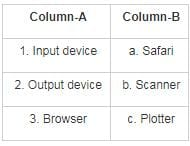Detailed Solution for RRB NTPC Mock Test - 15 (English) - Question 31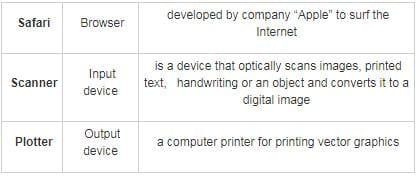RRB NTPC Mock Test - 15 (English) - Question 32

Find the sum of compound interest received when a sum of Rs. 25000 is invested at 5% p.a for 2 years and at 10 p.a for 1 year.

Detailed Solution for RRB NTPC Mock Test - 15 (English) - Question 32

Given,

⇒ Principal = P = 25000

⇒ Rate = R = 5%

⇒ Time = N = 2 years

⇒ Compound interest = [P{1 + R/100}n] – P = [25000{1 + (5/100)}2] – 25000 = 27562.5 – 25000 = 2562.5

Now,

⇒ Principal = P = 25000

⇒ Rate = R = 10%

⇒ Time = N = 1

⇒ Compound interest = [25000{1 + (10/100)}] – 25000 = 27500 – 25000 = 2500

⇒ Total compound interest = 2500 + 2562.5 = Rs. 5062.5

RRB NTPC Mock Test - 15 (English) - Question 33

Who was the architect of Jaipur City?

Detailed Solution for RRB NTPC Mock Test - 15 (English) - Question 33

Vidyadhar Bhattacharya was the chief architect and city planner of Jaipur Rajasthan.

RRB NTPC Mock Test - 15 (English) - Question 34

Alai Darwaja is a ______ to the enclosure of Quwat-Ul-Islam mosque at Delhi.

Detailed Solution for RRB NTPC Mock Test - 15 (English) - Question 34
• Alai Darwaja is a Gateway to the enclosure of Quwat-Ul-Islam mosque at Delhi.
• The Quwat-ul-Islam Mosque in the Qutab Minar complex was built by Alauddin Khilji, the Sultan of Delhi in 1311 AD.
• The Alai Darwaza has a domed entrance which was constructed using red sandstone.
RRB NTPC Mock Test - 15 (English) - Question 35

Who among the following is the present Chief Minister of Haryana?

Detailed Solution for RRB NTPC Mock Test - 15 (English) - Question 35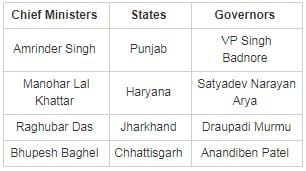RRB NTPC Mock Test - 15 (English) - Question 36

A palm tree and a mango tree of same height stand on either side of lake which is 80 m wide. Angle of elevations from a point somewhere in the lake are 60° and 45° respectively. What is the height of the trees?

Detailed Solution for RRB NTPC Mock Test - 15 (English) - Question 36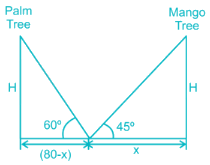From figure,

Let the height of Palm tree = AB = H units

⇒ Height of Mango tree = CD = H units

From figure,

⇒ PD = x units

⇒ BP = 80 – x units

Now, In triangle CPD,

⇒ tan 45° = CD/PD

⇒ 1 = H/X

⇒ H = X

Now, in triangle ABP,

⇒ tan 60° = AB/BP

⇒ √3 = H/(80 – X)

⇒ 80√3 – √3H = H

⇒ 138.56 = 2.732 H

⇒ H = 50.7 m

RRB NTPC Mock Test - 15 (English) - Question 37

A man had seven boys. When their average age was 14 years, a boy aged 14 years died. The average age of the remaining 6 boys is

Detailed Solution for RRB NTPC Mock Test - 15 (English) - Question 37

We know, Average of quantities = sum of all quantities/no. of quantities

The average age of 7 boys is 14 years. Then total age of 7 boys = 7 × 14 = 98 years.

A boy aged 14 years died, So now the total age of remaining 6 boys = 98 – 14 = 84 years.

∴ The average age of remaining 6 boys = 84/6 = 14 years.

RRB NTPC Mock Test - 15 (English) - Question 38

Which of the following is an indirect tax?

Detailed Solution for RRB NTPC Mock Test - 15 (English) - Question 38
• GK Trick: Trick to remember Direct & Indirect Taxes
Trick –– "Wepro, co, in (Direct Taxes)"
• We- Wealth Tax
• Pro- Property Tax
• Co- Corporate Tax
• In- Income Tax
Trick –– "Excuse Me (Indirect Taxes)"
• Ex- Excise tax
• Cu- Custom tax
• Se- Service tax
• M- Market tax/vat
• E- Entertainment tax
• Indirect tax refers to the tax that is levied on goods and services. Excise duty is a type of indirect tax that is levied on locally produced goods, company's revenue, and occupation.
• A direct tax is a type of tax that is levied on an individual or organization's income or profits. Capital Gains Tax, Wealth Tax, and Estate Duty are examples of direct tax.
RRB NTPC Mock Test - 15 (English) - Question 39

Fred starts from a point O. He turns North and walks 10 m. Then he turns right 90° and walks 5m. He then turns right 90° and walks 5 m. He then turns left 90° and walks 7 m.

Q. How far is Fred from his initial position?

Detailed Solution for RRB NTPC Mock Test - 15 (English) - Question 39

Note: For calculation purpose, various points are taken in the form of variables O, A, P, B, C and X.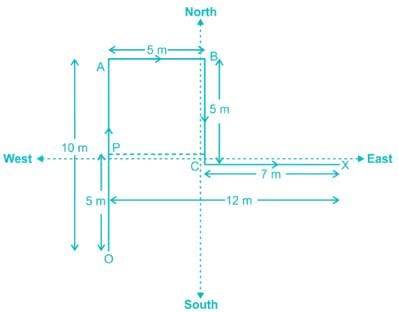We have to find the distance OX.

From the diagram we know that,

OA = 10 m

AB = 5 m

BC = 5 m

CX = 7 m

PC = AB = 5 m

PX = PC + CX = 12 m

AP = BC = 5 m

OP = OA – AP = 5 m

Using Pythagoras theorem in triangle OPX,

(OX)² = (OP)² + (PX)²

(OX)² = (5)² + (12)²

(OX)² = 25 + 144 = 169 m²

Hence OX = 13 m.

Hence, the distance between Fred’s initial position and the final position is 13 m.

RRB NTPC Mock Test - 15 (English) - Question 40

Find the HCF of 132 and 165.

Detailed Solution for RRB NTPC Mock Test - 15 (English) - Question 40

We can write,

⇒ 132 = 22 × 3 × 11

⇒ 165 = 3 × 5 × 11

∵ HCF is the product of the common factors

∴ HCF of 132 & 165 = 3 × 11 = 33

RRB NTPC Mock Test - 15 (English) - Question 41

Find the standard deviation of the series 9, 13, 15, 8, 6, 11, 7, 3, 10, 1.

Detailed Solution for RRB NTPC Mock Test - 15 (English) - Question 41

⇒ Mean of the series = (9 + 13 + 15 + 8 + 6 + 11 + 7 + 3 + 10 + 1)/10 = 83/10 = 8.3

Now,

⇒ (Standard deviation)2 = {(92 + 132 + 152 + 82 + 62 + 112 + 72 + 32 + 10+ 1)/10} – 8.32 = 85.5 – 68.89 = 16.61

⇒ Standard deviation = √16.61 = 4.07

RRB NTPC Mock Test - 15 (English) - Question 42

On which day the Indian Constitution came into force?

Detailed Solution for RRB NTPC Mock Test - 15 (English) - Question 42

The Indian Constitution came into force on Margsheersh Shukla Saptami, Samvat 2006 Vikrami.

• As per the English calendar, it came into force on 26th January 1950.
• It was adopted by the Constituent Assembly on 26th November 1949.
RRB NTPC Mock Test - 15 (English) - Question 43

India paid homage to Father of the Nation Mahatma Gandhi on his ________ death anniversary on 30th January 2019.

Detailed Solution for RRB NTPC Mock Test - 15 (English) - Question 43
• India is paying homage to Father of the Nation Mahatma Gandhi on his 71st death anniversary on 30th January, 2018.
• He was assassinated by Nathuram Godse on this day in 1948.
• The day is also observed as Martyrs' Day.
RRB NTPC Mock Test - 15 (English) - Question 44

Find the current (in A) when a charge of 500 Coulomb flows in 25 seconds.

Detailed Solution for RRB NTPC Mock Test - 15 (English) - Question 44

We know that, q =It

I =q/t

where

Current (I) = Charge (q)/Time (t)

Current = 500/25 = 20A

RRB NTPC Mock Test - 15 (English) - Question 45

{8 - (28 - 53)} ÷ {- 4 × 5 - (- 9)} = ?

Detailed Solution for RRB NTPC Mock Test - 15 (English) - Question 45

{8 - (28 - 53)} ÷ {- 4 × 5 - (- 9)} = {8 + 25} ÷ {- 20 + 9} = -33/11 = -3

RRB NTPC Mock Test - 15 (English) - Question 46

How many positive factors are there of 480?

Detailed Solution for RRB NTPC Mock Test - 15 (English) - Question 46

⇒ 480

⇒ 32 × 3 × 5

⇒ 25 × 31 × 51

∴ no. of positive factors of 480,

⇒ (5 + 1) × (1 + 1) × (1 + 1)

⇒ 24

RRB NTPC Mock Test - 15 (English) - Question 47

By the use of photovoltaic cell while converting solar energy which of the following is produced?

Detailed Solution for RRB NTPC Mock Test - 15 (English) - Question 47

Solar-powered photovoltaic (PV) panels convert the sun's rays into electricity by exciting electrons in silicon cells using the photons of light from the sun. This electricity can then be used to supply renewable energy to your home or business.

RRB NTPC Mock Test - 15 (English) - Question 48

The age of four family members is in the ratio 2 ∶ 3 ∶ 7 ∶ 8. If their average age is 25 years, find the difference between the age of the eldest and the youngest member of family.

Detailed Solution for RRB NTPC Mock Test - 15 (English) - Question 48

Let the age of 4 family members be ‘2x’, ‘3x’, ‘7x’ and ‘8x’ years

Now, average age = 25

⇒ (2x + 3x + 7x + 8x)/4 = 25

⇒ 20x = 100

⇒ x = 100/20 = 5

Hence,

⇒ Age of eldest member = 8x = 8(5) = 40 years

⇒ Age of youngest member = 2x = 2(5) = 10 years

∴ Required difference = 40 - 10 = 30 years

RRB NTPC Mock Test - 15 (English) - Question 49

The ratio of the amount that P and Q have is 5 ∶ 6. If P's amount is increased by 25% and Q's amount is decreased by 40%, and the average of the amount of P and Q is 5500. Find the ratio of the new amount of Q and P.

Detailed Solution for RRB NTPC Mock Test - 15 (English) - Question 49

Given,

Let earnings of P and Q be 5a and 6a respectively

Given,

⇒ Average = 5500

⇒ (5a + 6a)/2 = 5500

⇒ a = 1000

⇒ New earning of P = 5a + 25% of 5a = 5a × (125/100) = 25a/4 = 6250

⇒ New earning of Q = 6a – 40% of 6a = 6a × (60/100) = 18a/5 = 3600

⇒ Required ratio = 3600 ∶ 6250 = 72 ∶ 125

RRB NTPC Mock Test - 15 (English) - Question 50

Detailed Solution for RRB NTPC Mock Test - 15 (English) - Question 50
• The name of Madras State was changed in 1969 which was the result of The States Reorganization Act which was based on linguistic lines.
• The city of Madras was also renamed as Chennai subsequently.
• Before the name was changed Madras was a presidency and after changing name, it was given position of a state.
RRB NTPC Mock Test - 15 (English) - Question 51

The first trial run of Train 18 was conducted on Bareilly -________ railway section.

Detailed Solution for RRB NTPC Mock Test - 15 (English) - Question 51
• Train 18 has been named as Vande Bharat Express has now officially become India’s fastest train, which ran at a speed of 180 kilometers per hour during a trial run.
• The first trial run of the train was conducted on Bareilly-Moradabad railway section.
• Train 18 is India’s first indigenously built engine-less train.
• It was built by Chennai-based Integral Coach Factory (ICF).
• It will replace the premier Shatabdi Express.
RRB NTPC Mock Test - 15 (English) - Question 52

Who is the author of the book titled 'Where will man take Us? - A Bold story of the Man Technology is creating'?

Detailed Solution for RRB NTPC Mock Test - 15 (English) - Question 52
• Entrepreneur, technologist and science storyteller, Atul Jalan launched his new book titled, 'Where will man take Us? - A Bold story of the Man Technology is creating'.
• Authored by Atul Jalan, CEO of Manthan Systems, the book looks at the immediate future of mankind and what lies in wait.
RRB NTPC Mock Test - 15 (English) - Question 53

Rearrange the jumbled up letters in their natural sequence and find the odd one out.

Detailed Solution for RRB NTPC Mock Test - 15 (English) - Question 53

NAEGAMT → MAGENTA → a colour.

ITELVO → VIOLET → a colour.

LEWLOY → YELLOW → a colour.

RBAC → CRAB → an animal.

Therefore, RBAC is the odd one out.

RRB NTPC Mock Test - 15 (English) - Question 54

A series is given with one term missing. Select the correct alternative from the given ones that will complete the series.
1200, 360, 108, 32.4, ?, 2.916

Detailed Solution for RRB NTPC Mock Test - 15 (English) - Question 54

The given Series is: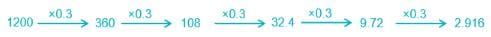Hence, the required term is 9.72.

RRB NTPC Mock Test - 15 (English) - Question 55

When a sound wave goes from one medium to another, which of the following quantity remains unchanged?

Detailed Solution for RRB NTPC Mock Test - 15 (English) - Question 55

When a sound wave goes from one medium into another, its frequency remains unchanged. The frequency of sound depends upon the source of the sound, not the medium of propagation. Hence, it does not change.

RRB NTPC Mock Test - 15 (English) - Question 56

In the figure given below, OD is the perpendicular bisector of the chord AB. If the diameter of the circle is 20 cm and the length of chord AB is 16 cm, find the length of CD.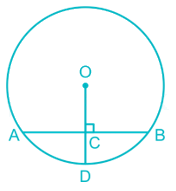Detailed Solution for RRB NTPC Mock Test - 15 (English) - Question 56

In the figure given below,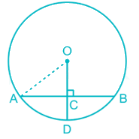As we know, the perpendicular bisector of a chord passes through the centre of the circle

⇒ OA = OB = OD = Radius of circle = 20/2 = 10 cm

⇒ AC = AB/2 = 16/2 = 8 cm

Now, applying Pythagoras theorem in ΔOCA,

⇒ OA2 = OC2 + AC2

⇒ 102 = OC2 + 82

⇒ OC2 = 100 – 64

⇒ OC = √36 = 6 cm

∴ CD = OD – OC = 10 – 6 = 4 cm

RRB NTPC Mock Test - 15 (English) - Question 57

Glass is soluble in

Detailed Solution for RRB NTPC Mock Test - 15 (English) - Question 57

Hydrofluoric acid is a solution of hydrogen fluoride (HF) in water. It is a colourless solution that is highly corrosive, capable of dissolving many materials, especially oxides. Its ability to dissolve glass has been known since the 17th century.

RRB NTPC Mock Test - 15 (English) - Question 58

In how many Lok Sabha constituencies did the voting begin in the first phase for the year 2019?

Detailed Solution for RRB NTPC Mock Test - 15 (English) - Question 58
• Voting began in 91 Lok Sabha constituencies spread across 18 states and 2 union territories in the first phase.
• In Odisha, voting for 28 out of 147 assembly seats and four Lok Sabha seats was also held on 11 April 2019.
• Voting for 543 Lok Sabha seats will be held in seven phases: April 11, April 18, April 23, April 29, May 6, May 12 and May 19. Counting will be done on May 23, 2019.
RRB NTPC Mock Test - 15 (English) - Question 59

Two years ago, the population of a village was 62500. If in two years, the population of the village increased by 24525, what is the rate of increase in population per year, if the rate of increase is same for both years?

Detailed Solution for RRB NTPC Mock Test - 15 (English) - Question 59

Let the rate of increase be ‘R%’

Population of village two years ago = 62500

Present population of village = 62500 + 24525 = 87025

∵ Population after n years = Population n years ago × (1 + Rate/100)n

Putting n = 2,

⇒ 87025 = 62500 × (1 + R/100)2

⇒ 1.3924 = (1 + R/100)2

⇒ √1.3924 = (1 + R/100)

⇒ 1.18 = 1 + R/100

⇒ R = 0.18 × 100 = 18%

∴ Rate of increase of population per year = 18%

RRB NTPC Mock Test - 15 (English) - Question 60

Which of the following Vedas has the mention of Saraswati River?

Detailed Solution for RRB NTPC Mock Test - 15 (English) - Question 60

Rigveda has the mention of Saraswati River in nadistuti hymn as the river that flows between the Yamuna in the east and the Sutlej in the west.

RRB NTPC Mock Test - 15 (English) - Question 61

A dishonest man marks up the price of goods by 25% and gives discount of 10%. But while selling he cheated by 150 gm per kg. Find percentage profit.

Detailed Solution for RRB NTPC Mock Test - 15 (English) - Question 61

Let CP of 1000 gm be Rs. 100

⇒ Marked price = 100 + 25% of 100 = 125

⇒ Discount = 10%

⇒ Selling Price of 850 gm = 125 – {125 × (10/100)} = 112.5

Now,

⇒ CP of 850gm = Rs. 85

⇒ Profit = Rs. (112.5 – 85) = Rs. 27.5

⇒ Profit percentage = (27.5/85) × 100 = 32.35%

RRB NTPC Mock Test - 15 (English) - Question 62

Panaji is the capital of which among the following states in India?

Detailed Solution for RRB NTPC Mock Test - 15 (English) - Question 62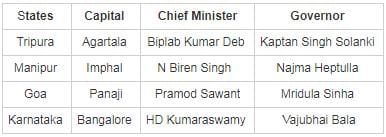RRB NTPC Mock Test - 15 (English) - Question 63

Direction: The table given below shows the marks obtained by four students in 5 different subjects. The marks are given out of 100. Read the table carefully and answer the following questions.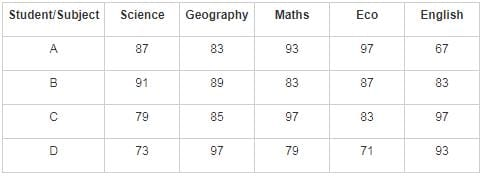Q. Which student got the highest marks in all the 5 subjects taken together?

Detailed Solution for RRB NTPC Mock Test - 15 (English) - Question 63

Marks scored by A in all the subjects = 427

Marks scored by B in all the subjects = 433

Marks scored by C in all the subjects = 441

Marks scored by D in all the subjects = 413

∴ Student C secured the highest marks in all the 5 subjects taken together.

RRB NTPC Mock Test - 15 (English) - Question 64

A train of length 150 m crosses a running person who is moving in the opposite direction in 20 seconds. If the speed of the train is 8 times the speed of the person, then the speed of the person is:

Detailed Solution for RRB NTPC Mock Test - 15 (English) - Question 64

Let speed of man be M m/s

⇒ Speed of train = 8M m/s

⇒ Relative speed = M + 8M = 9M m/s

⇒ Distance = 150 m

⇒ Speed = Distance/time

⇒ 9M = 150/20

⇒ M = 5/6 m/s

⇒ Speed of person = 5/6 m/s.

RRB NTPC Mock Test - 15 (English) - Question 65

Who was the first person to discover the pre-historic remains of Kalibangan?

Detailed Solution for RRB NTPC Mock Test - 15 (English) - Question 65

Luigi Pio Tessitori was the first person to discover the pre-historic remains of Kalibangan. He was an Italian Indologist who later sought help from Sir John Marshall of the Archaeological Survey of India.

RRB NTPC Mock Test - 15 (English) - Question 66

Who among the following has won a bronze medal at the Olympics held in the year 1952?

Detailed Solution for RRB NTPC Mock Test - 15 (English) - Question 66
• K.D. Jadhav has won a bronze medal at the Olympics held in the year 1952.
• He was the first Indian to win an individual Olympic medal in Wrestling that held at Helsinki.
• He was honoured with Arjuna Award in the year 2000.
• The government renamed the wrestling venue in the Indira Gandhi Sports Complex in New Delhi as the KD Jadhav Stadium in 2011.
RRB NTPC Mock Test - 15 (English) - Question 67

In a two digits number, the tenth digit is 5 more than its unit place digit. Product of number and difference between the ten's digit and unit digit is 470. Find 3/47th of the number.

Detailed Solution for RRB NTPC Mock Test - 15 (English) - Question 67

Let the two digits number be 10b + a

Given,

⇒ a – 5 = b

⇒ a – b = 5

Also,

⇒ (10b + a) × (b – a) = 470

⇒ 10b + a = 94

So, the number is 94

Now,

⇒ (3/47) × number = 94 × (3/47) = 6

∴ 3/47th of given number is 6

RRB NTPC Mock Test - 15 (English) - Question 68

Identify the odd one from the list below.

Detailed Solution for RRB NTPC Mock Test - 15 (English) - Question 68

Fern → a tree.

Bamboo → a grass.

Fig → a tree.

Palm → a tree.
Therefore, Bamboo is the odd one out.

Hence, Bamboo is the right answer.

RRB NTPC Mock Test - 15 (English) - Question 69

The ratio of the present ages of Mohan and Sohan is 2 ∶ 3 and 10 years from now, the ratio will get changed to 3 ∶ 4. What is the present age (in years) of Mohan?

Detailed Solution for RRB NTPC Mock Test - 15 (English) - Question 69

Let the present age of Mohan and Sohan be 2x and 3x

After 10 years, ratio of their ages will be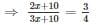⇒ 8x + 40 = 9x + 30

⇒ 9x - 8x = 40 - 30

⇒ x = 10

Hence, the present age of Mohan = 2x = 20 years

RRB NTPC Mock Test - 15 (English) - Question 70

In which among the following farming types in India, the produce is consumed by farmer and his family only?

Detailed Solution for RRB NTPC Mock Test - 15 (English) - Question 70
• In case of subsistence farming, the farmer produces crops for consumption of own family and there is no surplus to sell in the market.
• In Extensive Farming method, a limited amount of labour is used to cover a large area and machines are widely used.
• In Intensive Farming method, a large amount of labour is utilized for a very small area.
• In case of Plantation Agriculture, trees and bushes are planted on a large estate and a single crop such as rubber, coffee etc. is grown, mainly for exports.
RRB NTPC Mock Test - 15 (English) - Question 71

What is the mode of the following distribution?
1, 2, 3, 2, 2, 4, 5, 3, 3, 2, 2, 6

Detailed Solution for RRB NTPC Mock Test - 15 (English) - Question 71

We know that,

Mode refers to the most frequently occurring number in a set of numbers.

The given set of numbers is 1, 2, 3, 2, 2, 4, 5, 3, 3, 2, 2, 6

In the above set of numbers 2 appears most frequently i.e. 5 times.

∴ 2 is the mode of the given set of numbers.

RRB NTPC Mock Test - 15 (English) - Question 72

Direction: The Bar Graph shows the number of people in a theatre for 3 shows in a day. Study the graph carefully and answer the following questions.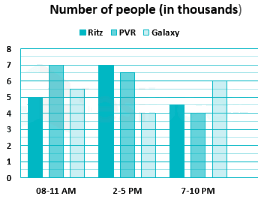Q. What is the ratio of people who watched all the shows in Ritz to the number of people who watched all the shows in PV

Detailed Solution for RRB NTPC Mock Test - 15 (English) - Question 72

Number of people who watched all the shows in Ritz = 16500

Number of people who watched all the shows in PVR = 17500

∴ Required ratio = 16500 ∶ 17500 = 33 ∶ 35

RRB NTPC Mock Test - 15 (English) - Question 73

A question is given followed by information in two statements. You have to find out the data in which statement(s) is sufficient to answer the question and mark your answer accordingly.

If the area of the Rhombus is 24 sq. cm then find the length of its diagonal?
I) Both diagonals cut each other at right-angle.
II) Both the diagonals are consecutive even numbers.

Detailed Solution for RRB NTPC Mock Test - 15 (English) - Question 73

Area of rhombus = 24 cm2

Let one diagonal be x cm and other be (x + 2) cm

Area = (1/2) × (d1 × d2)

⇒ 24 = (1/2) × (x × x + 2)

⇒ 48 = x2 + 2x

⇒ x2 + 2x - 48 = 0

⇒ x = 6, -8 (length cannot be negative)

Length of the diagonal is 6 cm and x + 2 = 8 cm

∴ Only II is sufficient.

RRB NTPC Mock Test - 15 (English) - Question 74

Pointing to a person Y, X says, “I am not the brother of my mother’s only daughter, but Y is the sister of my father’s mother’s only son”. How is Y related to X?

Detailed Solution for RRB NTPC Mock Test - 15 (English) - Question 74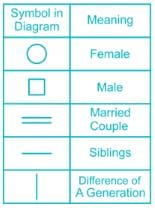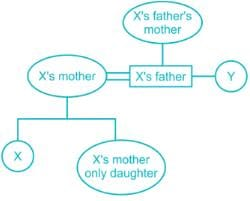Hence, Y is the paternal aunt of X.

RRB NTPC Mock Test - 15 (English) - Question 75

Radha buys an article at certain amount and marks it for sale at a price which gives her 75% profit on her cost price. She gave 20% discount on marked price. Calculate actual profit/loss percentage made by her.

Detailed Solution for RRB NTPC Mock Test - 15 (English) - Question 75

Let cost price of an article be Rs.100

⇒ Profit percentage = 75%

⇒ Marked price = 100 + 75% of 100 = Rs. 175

Now,

⇒ Discount percentage = 20%

⇒ Selling price after discount = 175 – 20% of 175 = Rs. 140

As,

⇒ Cost price = 100

⇒ Profit percentage = {(140 – 100)/100} × 100 = 40%

RRB NTPC Mock Test - 15 (English) - Question 76

The ratio of the speed of the boat to the speed of current is 5 : 2. If a person can row his boat 112 km downstream in 4 hours, find the speed of the boat in still water.

Detailed Solution for RRB NTPC Mock Test - 15 (English) - Question 76

SB : SC = 5 : 2

Speed of boat downstream, SD = 5 + 2 = 7 units = distance/time = 112/4 = 28 km/hr

⇒ 7 units = 28 km/hr

⇒ 1 units = 4 km/hr

∴ Speed of boat in still water, SB = 5 units = 5 × 4 = 20 km/hr

RRB NTPC Mock Test - 15 (English) - Question 77

What is the remainder when 221145 + 442522 is divided by 7.

Detailed Solution for RRB NTPC Mock Test - 15 (English) - Question 77

⇒ Remainder when 221 is divided by 7 = 4

⇒ Remainder when 442 is divided by 7 = 1

Now,

⇒ Rem {(221145 + 442522)/7} = Rem {(4145 + 1522)/7} = Rem {(4 + 1)/7} = Rem (5/7) = 5

∴ Remainder is 5

RRB NTPC Mock Test - 15 (English) - Question 78

Rearrange the jumbled up letters in their natural sequence and find the odd one out.

Detailed Solution for RRB NTPC Mock Test - 15 (English) - Question 78

AGO → GOA → a state of India.

HLDEI → DELHI → a union territory of India.

IKMSKI → SIKKIM → a state of India.

UAGAJRT → GUJARAT → a state of India.

Therefore, HLDEIis the odd one out.

RRB NTPC Mock Test - 15 (English) - Question 79

If the image below is rotated 90 degrees clockwise, then which of the answer figures is the right mirror image of the resulting figure?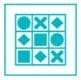Detailed Solution for RRB NTPC Mock Test - 15 (English) - Question 79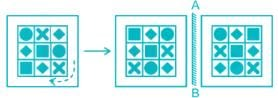Clearly, the correct right image is in option 1).

RRB NTPC Mock Test - 15 (English) - Question 80

From a 1 litre bottle filled with milk-water mixture in the ratio 7 ∶ 3, 60% of the mixture is poured out. Then, the bottle is filled with 100 ml honey, 200 ml milk and the remaining quantity with water. Find the ratio of milk and water in the final mixture in the bottle.

Detailed Solution for RRB NTPC Mock Test - 15 (English) - Question 80

⇒ Quantity of bottle = 1 litre = 1000 ml

After 60% of the mixture is poured out,

⇒ Quantity of mixture remaining in bottle = 40% of 1000 = 400 ml

⇒ Ratio of milk-water in the initial mixture = 7∶ 3

⇒ Quantity of milk in bottle = (7/10) × 400 = 280 ml

⇒ Quantity of water in bottle = (3/10) × 400 = 120 ml

Now,

⇒ Quantity of bottle to be filled = 1000 – 400 = 600 ml

⇒ Quantity of honey filled = 100 ml

⇒ Quantity of milk filled = 200 ml

⇒ Quantity of water filled = 600 – 100 – 200 = 300 ml

⇒ Quantity of milk in final mixture = 280 + 200 = 480 ml

⇒ Quantity of water in final mixture = 120 + 300 = 420 ml

⇒ Ratio of milk and water in final mixture = 480 ∶ 420 = 8 ∶ 7

RRB NTPC Mock Test - 15 (English) - Question 81

Directions: One statement is given, followed by two conclusions, I and II. You have to consider the statement to be true, even if it seems to be at variance from commonly known facts. You are to decide which of the given conclusions be definitely drawn from the given statement.

The statement: Cactus grows in the deserts which are usually dry.

Conclusions:
I.
Cactus plants require very less water to grow.
II. All deserts have Cactus plants.

Detailed Solution for RRB NTPC Mock Test - 15 (English) - Question 81

Cactus plants require less water to grow is a valid conclusion since it is the statement mentions that they grow in deserts which do not provide an ample amount of water to thrive. Hence conclusion I follows the statement. Coming to conclusion II, it doesn’t necessarily follow since the statement gives us no information that all deserts have Cactus plants. There might be deserts without any plantswhich require further conclusions apart from the statement.

RRB NTPC Mock Test - 15 (English) - Question 82

If ‘।’ means ‘minus’, ‘@’ means ‘plus’, ‘^’ means ‘multiplied by’ and ‘%’ means ‘divided by’, then find the value of
6 ^ 5 @ 76 । 34 % 2 =

Detailed Solution for RRB NTPC Mock Test - 15 (English) - Question 82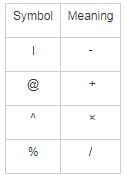Using BODMAS, we get

6 ^ 5 @ 76 । 34 % 2 = 6 × 5 + 76 - 34 / 2 = (6 × 5) + 76 - (34 / 2) = 30 + 76 – 17 = 89.

Hence, 89 is the correct answer.

RRB NTPC Mock Test - 15 (English) - Question 83

Which Indian state has the biggest network of rail routes in India?

Detailed Solution for RRB NTPC Mock Test - 15 (English) - Question 83
• Uttar Pradesh has the biggest network of rail routes in India.
• It has a total of 9077.45 kilometers of rail network.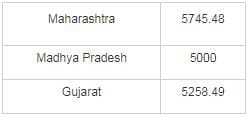RRB NTPC Mock Test - 15 (English) - Question 84

A box of chocolates consists of 30% milk chocolates and 45% are nuts chocolates. The remaining chocolates are filled with caramel. If there are 96 chocolates in the box, how many of them are caramel-filled chocolates?

Detailed Solution for RRB NTPC Mock Test - 15 (English) - Question 84

Percentage of caramel-filled chocolates = 100 - 30 - 45 = 25%

Total no. of chocolates = 96

∴ No. of caramel-filled chocolates = 25% of 96 = 1/4 × 96 = 24

RRB NTPC Mock Test - 15 (English) - Question 85

In the following question, by using which mathematical operators will the expression become correct?

If P = 3, Q = 14, R = 23, S = 4,T = 2, U = 13 and V = 2.

(P³ + Q - R × S ÷ T + U □ V) = 164.

Detailed Solution for RRB NTPC Mock Test - 15 (English) - Question 85

It is given that,

P = 3, Q = 14, R = 23, S = 4,T = 2, U = 13 and V = 2.

Given expression : (P3 + Q - R × S ÷ T + U □ V) = 164.

→ (33 + 14 – 23 × 4 ÷ 2 + 13 □ 2) = 164

→ (27 + 14 – 23 × 4 ÷ 2 + 13 □ 2) = 164

→ (27 + 14 – 23 × 2 + 13 □ 2) = 164

→ (27 + 14 – 46 + 13 □ 2) = 164

→ (– 5 + 13 □ 2) = 164

→ (13 □ 2) = 164 + 5 = 169

→ (13 □ 2) = 13 * 13 = 132

Only power operator can be used here to satisfy this equation.

→ 132 = 169

Hence, the power operator will be used to solve the equation.

RRB NTPC Mock Test - 15 (English) - Question 86

Find which of the answer figure can be formed from the pieces given in the question figure.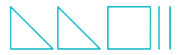Detailed Solution for RRB NTPC Mock Test - 15 (English) - Question 86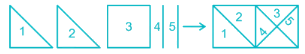Thus with all the given pieces, we can form the option 3 figure.

RRB NTPC Mock Test - 15 (English) - Question 87

In the following question, select the number which can be placed at the sign of the question mark (?) from the given alternatives.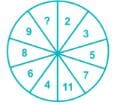Detailed Solution for RRB NTPC Mock Test - 15 (English) - Question 87

The pattern is:

In the figure, the consecutive prime numbers are opposite to consecutive non-prime numbers in increasing order.

2 is the first prime and it is opposite to the first non-prime 4.

3 is the second prime and it is opposite to the second non-prime 6.

5 is the third prime and it is opposite to the third non-prime 8.

7 is the fourth prime and it is opposite to the fourth non-prime 9.

Similarly,

11 is the fifth prime and it is opposite to the fifth non-prime 10.

Hence, the missing number in the figure is 10.

RRB NTPC Mock Test - 15 (English) - Question 88

There is a group of 6 people : A, B, C, D, E and F. A is taller than 3 people, but not taller than B. C is taller than D. C is taller than A and E. Who among the following can be the shortest person?

Detailed Solution for RRB NTPC Mock Test - 15 (English) - Question 88

People: A, B, C, D, E and F

1) A is taller than 3 people, but not taller than B.

→ B > A > 3 people

2) C is taller than D. C is taller than A and E. Hence only C and B are taller than A.

→ B, C > A > D, E

Hence, F is also shorter than A.

→ B, C > A > D, E, F

Hence, any one of D, E or F can be the shortest person.

RRB NTPC Mock Test - 15 (English) - Question 89

Which one set of letters when sequentially placed at the gaps in the given letter series shall complete it.

Z, B, X, _ , V, F, T, _

Detailed Solution for RRB NTPC Mock Test - 15 (English) - Question 89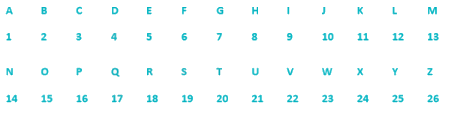The series is as follows:

The first letter from the end: Z

The second letter from the beginning: B

The third letter from the end: X

The fourth letter from the beginning: D

The fifth letter from the end: V

The sixth letter from the beginning: F

The seventh letter from the end: T

The eighth letter from the beginning: H

Hence, ‘D, H’ when placed at the gaps in the given letter series shall complete it.

RRB NTPC Mock Test - 15 (English) - Question 90

A series of figures is given which can be grouped into classes. Select the below correct option into which the figures can be classified from the given responses.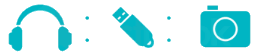Detailed Solution for RRB NTPC Mock Test - 15 (English) - Question 90

The figures represent a headset, a pen drive and a camera, all of which are electronic gadgets. Hence electronic gadgets is the correct answer.

RRB NTPC Mock Test - 15 (English) - Question 91

What is the change in the direction of light by a mirror called ______.

Detailed Solution for RRB NTPC Mock Test - 15 (English) - Question 91
• The process through which light rays falling on the surface of an object are sent back is called a reflection of light.
•  Thus, when light falls on the surface of an object it sends back the light.
• Reflection of light occurs when the waves encounter a surface or other boundary that does not absorb the energy of the radiation and bounces the waves away from the surface.
RRB NTPC Mock Test - 15 (English) - Question 92

Who is the ex-officio Chairman of Planning Commission?

Detailed Solution for RRB NTPC Mock Test - 15 (English) - Question 92
• The Prime Minister of India is the ex-officio Chairman of Planning Commission. Planning Commission was formed on 15 March 1950.
• On 01 January 2015, Planning Commission was replaced by another extra-constitutional body known as NITI (National Institution for Transforming India) Aayog.
• The Prime Minister is also the ex-officio Chairman of NITI Aayog.
RRB NTPC Mock Test - 15 (English) - Question 93

If [{b/(a – b)} + {a/(a + b)} + {2ab/(a2 – b2)}] = 8, find the value of a/b.

Detailed Solution for RRB NTPC Mock Test - 15 (English) - Question 93

Given,

⇒ [{b/(a – b)} + {a/(a + b)} + {2ab/(a2 – b2)}] = 8

We know that, (a + b)(a – b) = (a2 – b2)

⇒ {b(a + b) + a(a – b) + 2ab} / (a2 – b2) = 8

⇒ (ab + b2 + a2 – ab + 2ab) / (a2 – b2) = 8

⇒ (a2 + b2 + 2ab) / (a2 – b2) = 8

As, (a + b)2 = a2 + b2 + 2ab

⇒ (a + b)/ {(a + b)(a – b)} = 8

⇒ (a + b) / (a – b) = 8

⇒ a + b = 8a – 8b

⇒ 8a – a = b + 8b

⇒ 7a = 9b

∴ a/b = 9/7

RRB NTPC Mock Test - 15 (English) - Question 94

Cement is a result of which of the following?

Detailed Solution for RRB NTPC Mock Test - 15 (English) - Question 94

Cement is a binder substance that sets and hardens and can bind other materials together. It is formed out of the chemical reaction between Limestone and clay.

RRB NTPC Mock Test - 15 (English) - Question 95

Name a single letter that can be suffixed to the following words to form new words?
Glu, Nea, Sta, Cu

Detailed Solution for RRB NTPC Mock Test - 15 (English) - Question 95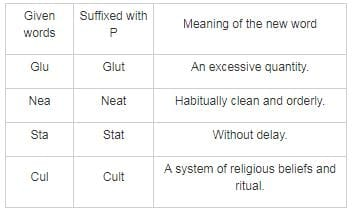Clearly, if the words are suffixed with T, meaningful words can be formed, otherwise, with the other letters, the words are meaningless.

RRB NTPC Mock Test - 15 (English) - Question 96

The chief constituent of water-glass is?

Detailed Solution for RRB NTPC Mock Test - 15 (English) - Question 96

Water-glass or liquid glass contains sodium silicate and these materials are available in aqueous solution and in solid form. They are used in cements, passive fire protection, textile and lumber processing, refractories, and automobiles.

RRB NTPC Mock Test - 15 (English) - Question 97

Directions: In the following questions are to be based on the details given below :

In a city of 300 people, 180 speak French. 20 people speak only Italian while 190 people don’t know Italian at all. 110 people speak exactly two languages and 60 people know both English and French. 30 people know only French and Italian.

Q. Which language is spoken by most people?

Detailed Solution for RRB NTPC Mock Test - 15 (English) - Question 97

We represent the whole scenario as a Venn diagram.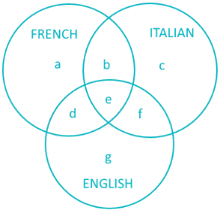Now according to the question:

Total population = a + b + c + d + e + f + g = 300.

People who speak French = a + b + d + e = 180.

People who only speak Italian = c = 20.

People who don’t know Italian = a + d + g = 190. Hence people who know Italian = b + c + e + f = 300 - 190 = 110.

People who speak exactly two languages = b + d + f = 110.

People who know both French and English = d + e = 60.

People who know only French and Italian = b = 30.

Since b + c + e + f = 110 and c = 20, then b + e + f = 90.

Now, b + e + f = 90 and b + d + f = 110. Subtracting, we get d - e = 20. Also, d + e = 60. Solving, we get d = 40 and e = 20.

Now, b = 30, d = 40, e = 20 and a + b + d + e = 180. Solving, we get a = 90.

Now b + d + f = 110, b = 30 and d = 40, hence f = 40.

Finally, g = 300 – (a + b + c + d + e + f) = 60.

Hence French is spoken by (a + b + d + e) = 180 people.

English is spoken by (d + e + f + g) = 160 people.

Italian is spoken by 110 people.

Hence French is the most popular.

RRB NTPC Mock Test - 15 (English) - Question 98

Directions: In the following questions are to be based on the details given below :

In a city of 300 people, 180 speak French. 20 people speak only Italian while 190 people don’t know Italian at all. 110 people speak exactly two languages and 60 people know both English and French. 30 people know only French and Italian.

Q. How many people speak all three languages?

Detailed Solution for RRB NTPC Mock Test - 15 (English) - Question 98

We represent the whole scenario as a Venn diagram.Now according to the question:

Total population = a + b + c + d + e + f + g = 300.

People who speak French = a + b + d + e = 180.

People who only speak Italian = c = 20.

People who don’t know Italian = a + d + g = 190. Hence people who know Italian = b + c + e + f = 300 - 190 = 110.

People who speak exactly two languages = b + d + f = 110.

People who know both French and English = d + e = 60.

People who know only French and Italian = b = 30.

Since b + c + e + f = 110 and c = 20, then b + e + f = 90.

Now, b + e + f = 90 and b + d + f = 110. Subtracting, we get d - e = 20. Also, d + e = 60. Solving, we get d = 40 and e = 20.

Now, b = 30, d = 40, e = 20 and a + b + d + e = 180. Solving, we get a = 90.

Now b + d + f = 110, b = 30 and d = 40, hence f = 40.

Finally, g = 300 – (a + b + c + d + e + f) = 60.

Hence French is spoken by (a + b + d + e) = 180 people.

English is spoken by (d + e + f + g) = 160 people.

Italian is spoken by 110 people.

Hence 20 people speak all three languages.

RRB NTPC Mock Test - 15 (English) - Question 99

Directions: In the following questions are to be based on the details given below :

In a city of 300 people, 180 speak French. 20 people speak only Italian while 190 people don’t know Italian at all. 110 people speak exactly two languages and 60 people know both English and French. 30 people know only French and Italian.

Q. How many people know English but no other language?

Detailed Solution for RRB NTPC Mock Test - 15 (English) - Question 99

We represent the whole scenario as a Venn diagram.Now according to the question, we need to find g:

Total population = a + b + c + d + e + f + g = 300.

People who speak French = a + b + d + e = 180.

People who only speak Italian = c = 20.

People who don’t know Italian = 190. Hence people who know Italian = b + c + e + f = 300 - 190 = 110.

People who speak exactly two languages = b + d + f = 110.

People who know both French and English = d + e = 60.

People who know only French and Italian = b = 30.

Since b + c + e + f = 110 and c = 20, then b + e + f = 90.

Now, b + e + f = 90 and b + d + f = 110. Subtracting, we get d - e = 20. Also, d + e = 60. Solving, we get d = 40 and e = 20.

Now, b = 30, d = 40, e = 20 and a + b + d + e = 180. Solving, we get a = 90.

Now b + d + f = 110, b = 30 and d = 40, hence f = 40.

Finally, g = 300 – (a + b + c + d + e + f) = 60.

Hence English alone is spoken by 60 people.

RRB NTPC Mock Test - 15 (English) - Question 100

Directions: In the following questions are to be based on the details given below :

In a city of 300 people, 180 speak French. 20 people speak only Italian while 190 people don’t know Italian at all. 110 people speak exactly two languages and 60 people know both English and French. 30 people know only French and Italian.

Q. How many people know French but no other language?

Detailed Solution for RRB NTPC Mock Test - 15 (English) - Question 100

We represent the whole scenario as a Venn diagram.Now according to the question, we need to find a :

Total population = a + b + c + d + e + f + g = 300.

People who speak French = a + b + d + e = 180.

People who only speak Italian = c = 20.

People who don’t know Italian = 190. Hence people who know Italian = b + c + e + f = 300 - 190 = 110.

People who speak exactly two languages = b + d + f = 110.

People who know both French and English = d + e = 60.

People who know only French and Italian = b = 30.

Since b + c + e + f = 110 and c = 20, then b + e + f = 90.

Now, b + e + f = 90 and b + d + f = 110. Subtracting, we get d - e = 20. Also, d + e = 60. Solving, we get d = 40 and e = 20.

Now, b = 30, d = 40, e = 20 and a + b + d + e = 180. Solving, we get a = 90.

Hence French alone is spoken by 90 people.

## RRB NTPC - Mock Tests Papers 2020

20 tests
 Use Code STAYHOME200 and get INR 200 additional OFF Use Coupon Code
Information about RRB NTPC Mock Test - 15 (English) Page
In this test you can find the Exam questions for RRB NTPC Mock Test - 15 (English) solved & explained in the simplest way possible. Besides giving Questions and answers for RRB NTPC Mock Test - 15 (English), EduRev gives you an ample number of Online tests for practice

20 tests